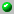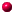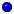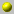Andrew Mathas

The homepage can be accessed here.

Andrew Mathas

the following papers are available on our server= dvi format= ps format= tex format= pdf formata_q_analogue_of_the_coxeter_complexa_q_analogue_of_the_jantzen_schaper_thoeremcanonical_bases_and_the_decomposition_matrices_of_ariki_koike_algebrascyclotomic_q_schur_algebraselementary_divisors_of_specht_moduleshecke_algebras_and_schur_algebras_of_the_symmetric_grouphecke_algebras_of_type_a_with_q_=_-1murphy_operators_and_the_centre_of_the_iwahore_hecke_algebras_of_type_aon_the_left_cell_representations_of_iwahori_hecke_algenras_of_finite_coxeter_groupssimple_modules_of_ariki_koike_algebrassome_generic_representations_w_graphs_and_dualitysymmetric_cyclotomic_hecke_algebrasthe_cyclotomic_jantzen_schaper_theoremthe_irreducible_specht_modules_in_characteristic_2the_(Q,q)_schur_algebra

Go back to the directory of pre and reprints.
Or search the database by keywords
Or look for the newest entires in the database